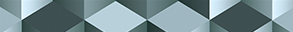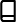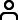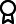Journal metrics
See full report
Acceptance rate30%
Submission to final decision59 days
Acceptance to publication22 days
CiteScore2.600
Journal Citation Indicator1.430
Impact Factor1.9

### The Existence and Multiplicity of Solutions for -Laplacian-Like Neumann Problems

####Journal profile

Journal of Function Spaces publishes research on all aspects of function spaces, functional analysis, and their employment across other mathematical disciplines.

####Editor spotlight

Chief Editor, Dr Ragusa, is a full professor of mathematical analysis at University of Catania, Italy. Her research interests include partial differential equations and real analysis.

####Special Issues

We currently have a number of Special Issues open for submission. Special Issues highlight emerging areas of research within a field, or provide a venue for a deeper investigation into an existing research area.

## Latest Articles

More articles
Research Article

### Ground State Solutions of Schrödinger-Kirchhoff Equations with Potentials Vanishing at Infinity

In this paper, we deal with the following Schrödinger-Kirchhoff equation with potentials vanishing at infinity: and where and with , and . We first prove the existence of positive ground state solutions under the assumption that for some , then we show that concentrates at a global minimum point of .

Research Article

### Analysis of Option Butterfly Portfolio Models Based on Nonparametric Estimation Deep Learning Method

The option butterfly portfolio is the commonly option arbitrage strategy. In reality, because the distribution of the option state price density (SPD) function is not normal and unknown, so the nonparametric deep learning methods to estimate option butterfly portfolio returns are proposed. This paper constructs the single-index nonparametric option pricing model which contains multiple influencing factors and presents the nonparametric estimation form for option butterfly portfolio returns. The empirical analysis shows that the SPD function estimated by using single-index nonparametric option model can effectively calculate the option butterfly portfolio returns with the minimum option strike price interval and provide an effective reference tool for risk-averse investors with limited risk preferences.

Research Article

### Applications and Properties for Bivariate Bell-Based Frobenius-Type Eulerian Polynomials

In this study, we introduce sine and cosine Bell-based Frobenius-type Eulerian polynomials, and by presenting several relations and applications, we analyze certain properties. Our first step is to obtain diverse relations and formulas that cover summation formulas, addition formulas, relations with earlier polynomials in the literature, and differentiation rules. Finally, after determining the first few zero values of the Eulerian polynomials, we draw graphical representations of these zero values.

Research Article

### Some Interesting Inequalities for the Class of Generalized Convex Functions of Higher Order

In this paper, we study a generalized version of strongly reciprocally convex functions of higher order. Firstly, we prove some basic properties for addition, scalar multiplication, and composition of functions. Secondly, we establish Hermite-Hadamard and Fejér type inequalities for the generalized version of strongly reciprocally convex functions of higher order. We also include some fractional integral inequalities concerning with this class of functions. Our results have applications in optimization theory and can be considered extension/generalization of many existing results.

Research Article

### Newfangled Linearization Formula of Certain Nonsymmetric Jacobi Polynomials: Numerical Treatment of Nonlinear Fisher’s Equation

This article is devoted to deriving a new linearization formula of a class for Jacobi polynomials that generalizes the third-kind Chebyshev polynomials class. In fact, this new linearization formula generalizes some existing ones in the literature. The derivation of this formula is based on employing a new moment formula of this class of polynomials and after that using suitable symbolic computation to reduce the resulting linearization coefficients into simplified forms that do not contain any hypergeometric functions or sums. The new formula is employed along with some other formulas and with the utilization of the spectral tau method to obtain numerical solutions to the nonlinear Fisher equation. The presented method is used to convert the equation governed by its underlying conditions into a nonlinear system of equations. The solution of the resulting system can be obtained through any suitable standard numerical scheme. To demonstrate the efficiency and usefulness of the proposed algorithm, some examples are shown, including comparisons with some existing techniques in the literature.

Research Article

### Complete Continuity of Composition-Differentiation Operators on the Hardy Space

We study composition-differentiation operators on the Hardy space on the unit disk. We prove that if is an analytic self-map of the unit disk such that the composition-differentiation operator induced by is bounded on the Hardy space , then it is completely continuous. This result is stronger than the similar result for composition operators which says that the composition operator induced by is completely continuous if and only if almost everywhere on the unit circle.Journal metrics
See full report
Acceptance rate30%
Submission to final decision59 days
Acceptance to publication22 days
CiteScore2.600
Journal Citation Indicator1.430
Impact Factor1.9Author guidelinesEditorial boardDatabases and indexingAuthor guidelinesEditorial boardDatabases and indexing

Article of the Year Award: Impactful research contributions of 2022, as selected by our Chief Editors. Discover the winning articles.